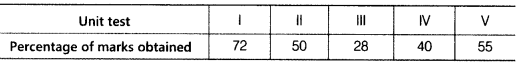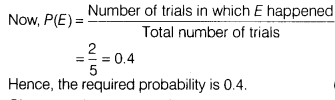# The percentage of marks obtained by a student in five unit tests are given below

The percentage of marks obtained by a student in five unit tests are given belowA unit test is’selected at random. What is the probability that the student gets more than 50% marks in the test?

Given, total number of unit tests = 5
∴ Total number of trials = 5
Let E be the event of getting a unit test in which a student gets more than 50% marks.
∴ Number of trials in which the event E happened = 2